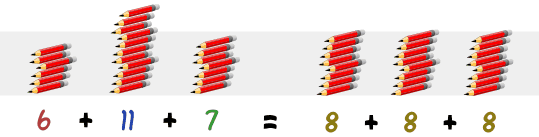# Statistics

How to Find the Mean

The mean is just the average of the numbers.

It is easy to calculate: add up all the numbers, then divide by how many numbers there are.

———————————————————————————————————

Example 1:
What is the Mean of these numbers?
6, 11, 7

Add the numbers: 6 + 11 + 7 = 24
Divide by how many numbers (there are 3 numbers): 24 / 3 = 8

The Mean is 8

———————————————————————————————————-

Why Does This Work?

It is because 6, 11 and 7 added together is the same as 3 lots of 8:It’s like you are “flattening out” the numbers

.
———————————————————————————————————-

Example 2:
Look at these numbers:

3, 7, 5, 13, 20, 23, 39, 23, 40, 23, 14, 12, 56, 23, 29

The sum of these numbers is 330

There are fifteen numbers.

The mean is equal to 330 / 15 = 22
The mean of the above numbers is 22

————————————————————————————————————

Negative Numbers

How do you handle negative numbers? Adding a negative number is the same as subtracting the number (without the negative). For example 3 + (-2) = 3-2 = 1.

Knowing this, let us try an example:

————————————————————————————————————-
Example 3:

Find the mean of these numbers:

3, -7, 5, 13, -2

* The sum of these numbers is 3 – 7 + 5 + 13 – 2 = 12
* There are 5 numbers.
* The mean is equal to 12 ÷ 5 = 2.4

The mean of the above numbers is 2.4

___________________________________________________________________________________________________

## How to Find the Median Value

It’s the middle number in a sorted list.

How to Find the Median Value

To find the Median, place the numbers you are given in value order and find the middle number.

————————————————————————————————————

Example 1:

find the Median of {12, 3 and 5}

Put them in order:

3, 5, 12

The middle number is 5, so the median is 5.

————————————————————————————————————

Example 2

Look at these numbers:

3, 13, 7, 5, 21, 23, 39, 23, 40, 23, 14, 12, 56, 23, 29

If we put those numbers in order we have:

3, 5, 7, 12, 13, 14, 21, 23, 23, 23, 23, 29, 39, 40, 56

There are fifteen numbers. Our middle number will be the eighth number:

3, 5, 7, 12, 13, 14, 21, 23, 23, 23, 23, 29, 39, 40, 56

The median value of this set of numbers is 23.

(Note that it didn’t matter if we had some numbers the same in the list)

————————————————————————————————————

Two Numbers in the Middle

BUT, if there are an even amount of numbers things are slightly different.

In that case we need to find the middle pair of numbers, and then find the value that would be half way between them. This is easily done by adding them together and dividing by two.

An example will help:
3, 13, 7, 5, 21, 23, 23, 40, 23, 14, 12, 56, 23, 29

If we put those numbers in order we have:

3, 5, 7, 12, 13, 14, 21, 23, 23, 23, 23, 29, 40, 56

There are now fourteen numbers and so we don’t have just one middle number, we have a pair of middle numbers:

3, 5, 7, 12, 13, 14, 21, 23, 23, 23, 23, 29, 40, 56

In this example the middle numbers are 21 and 23.

To find the value half-way between them, add them together and divide by 2:

21 + 23 = 44
44 ÷ 2 = 22

And, so, the Median in this example is 22.

(Note that 22 was not in the list of numbers … but that is OK, because half the numbers in the list are less, and half the numbers are greater.)

Advertisements